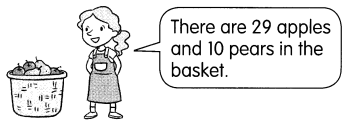# Math in Focus Grade 1 Chapter 14 Practice 2 Answer Key Mental Subtraction

Practice the problems of Math in Focus Grade 1 Workbook Answer Key Chapter 14 Practice 2 Mental Subtraction to score better marks in the exam.

## Math in Focus Grade 1 Chapter 14 Practice 2 Answer Key Mental Subtraction

Question 1.
8 – 5 = ______

Question 2.
9 – 6 = ______

Question 3.
11 – 3 = ______

Question 4.
13 – 7 = ______

Question 5.
15 – 6 = ______

Question 6.
12 – 8 = ______

Subtract mentally. First subtract the tens. Then add the tens to the ones.

Example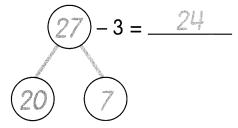Question 7.
28 – 4 = ___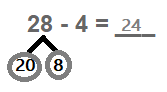Question 8.
29 – 5 = ______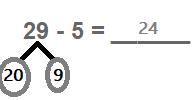Question 9.
27 – 6 = ______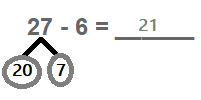Question 10.
37 – 2 = ______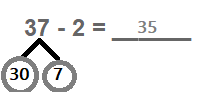Question 11.
38 – 8 = ______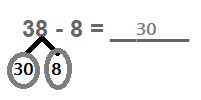Question 12.
36 – 6 = ______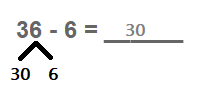Subtract mentally.
First subtract the tens.
Then add the tens to the ones.

Example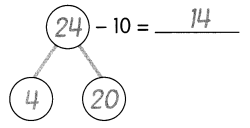Question 13.
22 – 10 = _______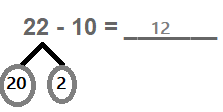Question 14.
23 – 20 = _______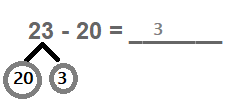Question 15.
35 – 30 = ________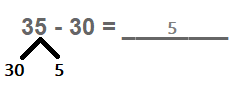Question 16.
36 – 20 = ________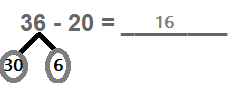Solve mentally. Fill in the blanks.

Question 17.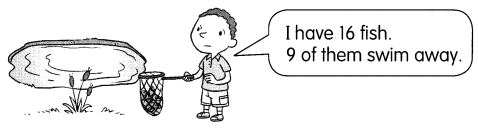How many fish are left? ______